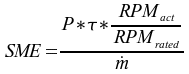Specific Mechanical Energy

Specific Mechanical Energy (SME) is a scale-independent measure of the mechanical energy put into the extrudate.

The basic concept behind SME is to measure the energy going into the extrusion system per unit mass in the form of work from the motor. That energy is put into the extrudate through viscous dissipation. That energy is converted primarily into heat in the extruder. There are other reactions that can occur, such as gelatinization of starch or denaturation of protein and some, of course, is lost across the boundary (through the barrel wall) of the extruder. Unfortunately, there are a lot of uncertainties in measuring the mechanical input, so getting an extremely accurate SME is somewhat uncertain.

One of the more straight-forward SME calculations is as follows:where:

P = Motor power, expressed in kW

τ = Motor Load or Torque, expressed as a decimal expression of the rated power. Strictly speaking, this should be the running torque minus the torque when the extruder is running empty.

RPMact = the actual RPM of the extruder

RPMrated = the rated RPM of the extruder, that is to say the extruder RPM when the motor is running at it's rated speed (rated motor speed/gear ratio in the gearbox or speed reduction in the pulley drive system)

ṁ = the mass flow rate of the system (kg/sec).

The output of the calculation will be in kJ/kg.  Assuming you know the heat capacity of the extrudate, the temperature rise can be calculated from this.

A more full calculation of this type can be found in the Appendix (page 211 for info on SME) of “Extruders in Food Applications”, edited by Mian Riaz.

There are a lot of caveats that go with this calculation. The ultimate goal of this page is to catalog the SME calculations that are commonly used and (if possible), follow the calculations back to the calculation of energy delivery of an electric motor.

One caveat that can be noted immediately is that there are a variety of views on what the mass flow rate entails.  The "easiest" calculation is to assume that the mass flow rate consists only of the dry feed.  That gives a measure of work done on the dry feed that would be independent of the water content of the system, a measure of mechanical manipulation/damage performed on the dry extrudate.  The less easy calculation is to include the entire mass flow rate (dry feed + preconditioner water + preconditioner steam + barrel water + barrel steam + any other mass entering the system.  As this measure is used to compare one system to another and/or to calculate final extrudate temperatures, it tends to be unimportant which form of the equation is chosen.  It becomes of critical importance to communicate how SME is measured when reporting it to other people.